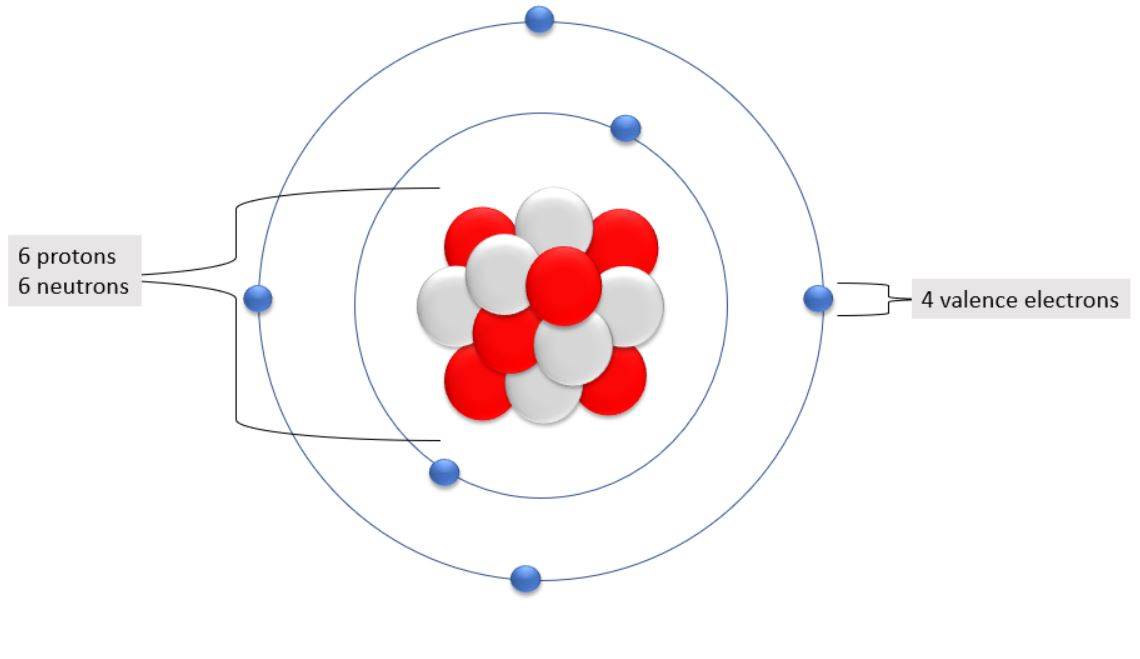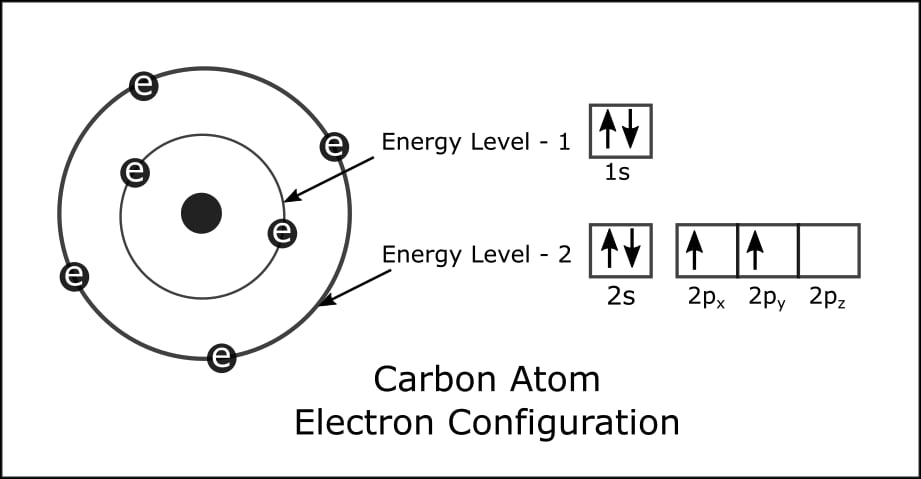# How Many Valence Electrons Does Carbon Have?

Carbon is the sixth element in the periodic table and the first element in Group 14 of the periodic table. Carbon has an atomic mass of 12.0096 and the symbol ‘C.’ Carbon takes part in bond formation via valence electrons. Read through to get more information on How Many Valence Electrons Does Carbon Have?### How Many Electrons, Protons, and Neutrons does a Carbon Atom have?

The nucleus is at the heart of the atom. The nucleus contains protons and neutrons. Carbon has an atomic number of 6. The atomic number represents the number of protons.

There are six protons in carbon. They arranged protons and electrons in a circular shell outside the nucleus. A carbon atom possesses six electrons in total.

They calculate the number of neutrons in an element by subtracting the number of atomic masses from the number of atoms. To put it another way, the neutron number (n) = atomic mass number (A) – atomic number (Z)

Carbon has an atomic number of 6 and an atomic mass number of 12. 12 – 6 = 6 neutrons (n). Therefore, the number of neutrons in carbon is 6.

### How Many Valence Electrons Does Carbon Have?

The valence electrons of carbon are the total number of electrons in the last shell after the electron configuration of carbon.

The total number of electrons in the last orbit is referred to as the valence electron. The valence electrons determine the characteristics of the element and play a role in bond formation.

### How do you Calculate the Number of Valence Electrons in a Carbon Atom?

They must take a few steps to find out the valence electrons. One of them is the electron configuration. The valence electron cannot be determined in the absence of electron configuration.

Knowing the electron configuration correctly makes determining the valence electrons of all elements relatively simple.

Valence electrons can be easily identified by arranging electrons according to the Bohr principle. We will now learn how to quickly determine the valence electrons of carbon.

#### 1. Determining the Total number of Electrons in Carbon

To begin, we must determine the total number of electrons in the carbon atom. For you to calculate the number of electrons, first determine the number of protons in carbon.

In order to get the number of protons, you must first determine the atomic number of the carbon element. A periodic table is required to determine the atomic number.

The periodic table must determine the atomic number of carbon elements.

#### 2. Do Electron Configuration of Carbon

The second step is critical. Carbon electrons must be organized in this stage. Carbon atoms have six electrons.

Carbon’s electron arrangement reveals two electrons in the K shell and four in the L shell. That example, the first shell of carbon has two electrons, while the second shell has four.

### Determine the Valence Shell and Calculate the Total ElectronsThey must diagnose the valence shell in the third phase. The valence shell is the shell that comes after the electron configuration. A valence electron is the total number of electrons in a valence shell.

Carbon’s electron arrangement reveals that the last shell of carbon has four electrons (2s2 2p2). As a result, carbon has four valence electrons.

### What is the Valency of Carbon?

Valency is the capacity of one atom of an element to join another atom during the creation of a molecule (valence).

The number of unpaired electrons in an element’s final orbit determines its valency. Carbon’s proper electron structure in ground state is 1s2 2s2 2px1 2py1.

Carbon’s electron structure reveals two unpaired electrons. The valency of the carbon atom in this example is 2. In addition, electron configuration in the excited state determines an element’s valency.

### Ionic Properties of Carbon Atoms

When a charge-neutral atom receives an electron and turns it into a negative ion, it is called an anion. The last orbit of a carbon atom has four electrons.

The carbon atom first takes two electrons and then 2 more electrons to fill the octave and become an anion.

### Compound Formation of Carbon by Valence Electrons

Carbon takes part in bond formation via its valence electrons. We know that carbon has four valence electrons. This valence electron is involved in creating bonds with other elemental atoms.

Carbon atoms create bonds with hydrogen atoms by exchanging electrons. The electron configuration of hydrogen shows that hydrogen only contains one electron.

By sharing electrons, four hydrogen atoms and one carbon atom form methane(CH4) molecules. As a result, the carbon atom completes its octave and gains the neon electron state.

## Frequently Asked Questions

1. Does Carbon have 4 or 8 Valence Electrons?

The electrons present in the outermost shell are called valence electrons. Carbon has four valence electrons and here a valence of carbon is four.

2. Does Every Carbon have 4 Valence Electrons?
Because each carbon is identical, they all have four valence electrons, so they can easily bond with other carbon atoms to form long chains or rings.

3. Does Carbon have 4 or 6 Valence Electrons?
The electrons present in the outermost shell are called valence electrons. Carbon has four valence electrons and here a valence of carbon is four.

4. Does Carbon have 6 Valence Electrons?
There are 4 valance electrons in Carbon.

5. Does Carbon have 2 or 4 Valence Electrons?

The electronic configuration of carbon is 2,4. So, it forms mainly covalent compound as it can neither lose nor gain four-electron to complete its octet. The example of such compound is Methane (CH4).

### More Frequently Asked Questions

6. Why does Carbon have 2 and 4 Valence Electrons?
By forming four covalent bonds, carbon shares four pairs of electrons, thus filling its outer energy level and achieving stability.

7. Why is Carbon Valency 4?

Now, we know that the number of electrons in the outermost shell of carbon is 4. In order to achieve the noble gas configuration of the nearest noble gas, carbon makes four bonds with hydrogen. Therefore, the valency of carbon is 4. It is also called the tetravalency of carbon.

8. Why does Carbon Show Valency 4?

A carbon atom contains 4 electrons in its outermost shell. So, its covalency is 4.

Valence electrons are the total number of electrons in an atom’s outermost shell (i.e. in the outermost orbital).

A neutral atom’s valence electrons are always definite; they cannot be changed under any condition for a specific atom and may not be equal to its valency.

Mostly, valency varies/changes because of changes in oxidation and reduction states.